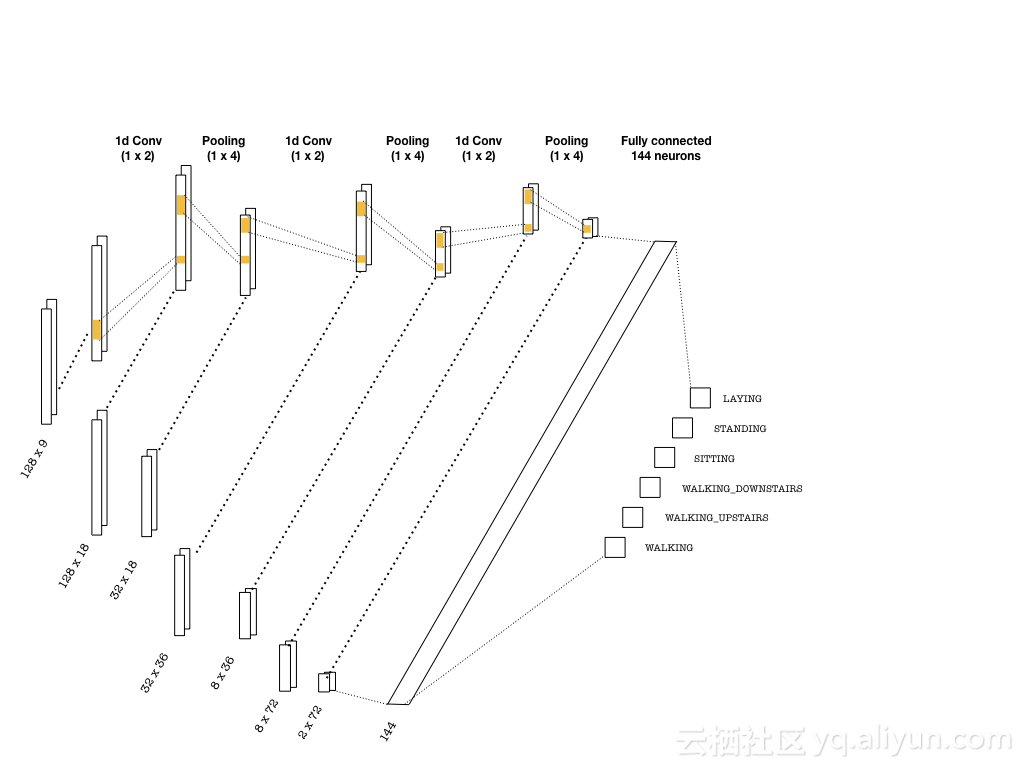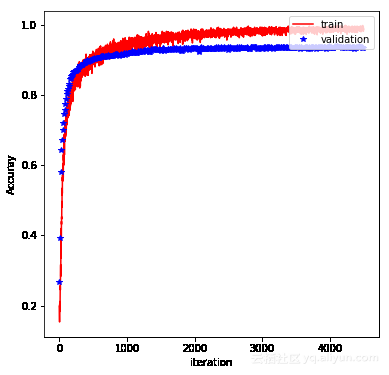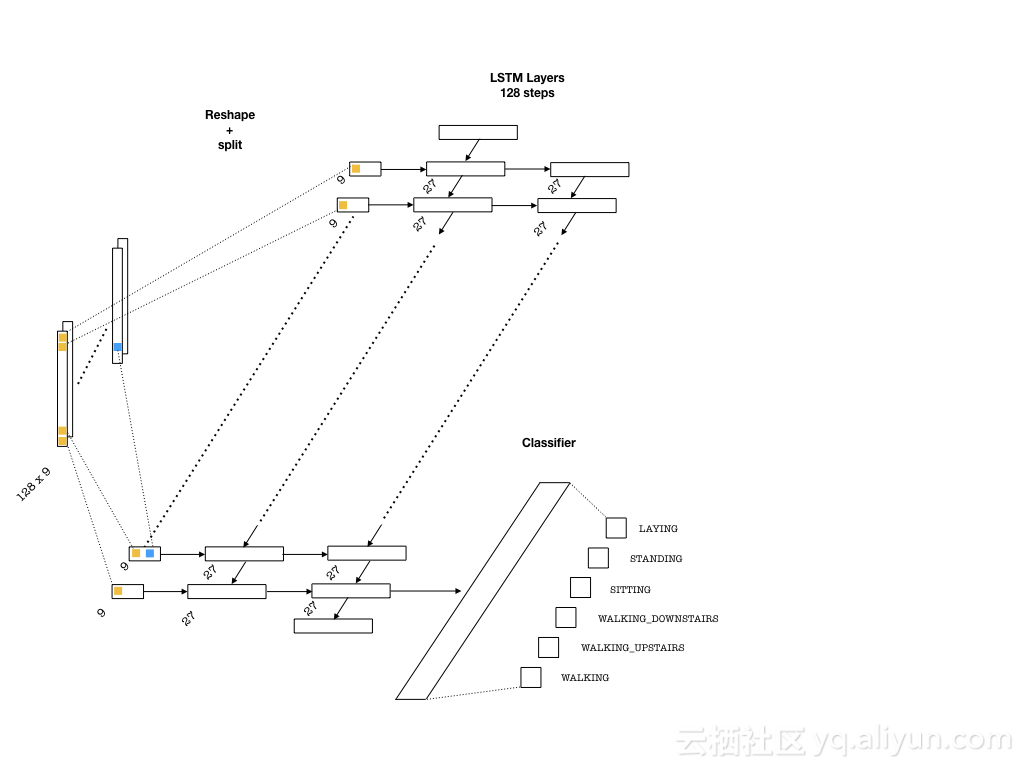#0
0
01. 云栖社区>
2. 博客>
3. 正文

## 如何使用最流行框架Tensorflow进行时间序列分析——第二篇

``````graph = tf.Graph()
with graph.as_default():
inputs_ = tf.placeholder(tf.float32, [None, seq_len, n_channels],name = 'inputs')
labels_ = tf.placeholder(tf.float32, [None, n_classes], name = 'labels')
keep_prob_ = tf.placeholder(tf.float32, name = 'keep')
learning_rate_ = tf.placeholder(tf.float32, name = 'learning_rate')
``````

`inputs是将`输入的张量馈送到计算图，并将其数组第一个位置设置为`None``，以便`允许可变的批量大小。`labels_`是要预测的一个热编码的标签，`keep_prob``的作用`是在退出正则化中保持概率来防止过度拟合，并且`learning_rate_`Adam优化器中使用的学习率。``````with graph.as_default():
# (batch, 128, 9) -> (batch, 32, 18)
conv1 = tf.layers.conv1d(inputs=inputs_, filters=18, kernel_size=2, strides=1,padding='same', activation = tf.nn.relu)
max_pool_1 = tf.layers.max_pooling1d(inputs=conv1, pool_size=4, strides=4, padding='same')
# (batch, 32, 18) -> (batch, 8, 36)
conv2 = tf.layers.conv1d(inputs=max_pool_1, filters=36, kernel_size=2, strides=1,padding='same', activation = tf.nn.relu)
max_pool_2 = tf.layers.max_pooling1d(inputs=conv2, pool_size=4, strides=4, padding='same')
# (batch, 8, 36) -> (batch, 2, 72)
conv3 = tf.layers.conv1d(inputs=max_pool_2, filters=72, kernel_size=2, strides=1,padding='same', activation = tf.nn.relu)
max_pool_3 = tf.layers.max_pooling1d(inputs=conv3, pool_size=4, strides=4, padding='same')
``````

1.    计算softmax交叉熵，这是多类问题中使用的标准损失度量。

2.    从最大概率以及精度预测类标签。

``````with graph.as_default():
flat = tf.reshape(max_pool_3, (-1, 2*72))
flat = tf.nn.dropout(flat, keep_prob=keep_prob_)
# Predictions
logits = tf.layers.dense(flat, n_classes)
# Cost function and optimizer
cost =        tf.reduce_mean(tf.nn.softmax_cross_entropy_with_logits(logits=logits,labels=labels_))
# Accuracy
correct_pred = tf.equal(tf.argmax(logits, 1), tf.argmax(labels_, 1))
accuracy = tf.reduce_mean(tf.cast(correct_pred, tf.float32), name='accuracy')
``````## 长短期记忆网络（LSTM）

LSTM在处理基于文本的数据方面非常受欢迎，在情感分析，语言翻译和文本生成方面也相当成功。今天我们就用LSTM来解决我们今天的问题。``````with graph.as_default():
# Construct the LSTM inputs and LSTM cells
lstm_in = tf.transpose(inputs_, [1,0,2]) # reshape into (seq_len, N, channels)
lstm_in = tf.reshape(lstm_in, [-1, n_channels]) # Now (seq_len*N, n_channels)
# To cells
lstm_in = tf.layers.dense(lstm_in, lstm_size, activation=None)
# Open up the tensor into a list of seq_len pieces
lstm_in = tf.split(lstm_in, seq_len, 0)
lstm = tf.contrib.rnn.BasicLSTMCell(lstm_size)
drop = tf.contrib.rnn.DropoutWrapper(lstm, output_keep_prob=keep_prob_)
cell = tf.contrib.rnn.MultiRNNCell([drop] * lstm_layers)
initial_state = cell.zero_state(batch_size, tf.float32)
``````

``````with graph.as_default():
outputs, final_state = tf.contrib.rnn.static_rnn(cell, lstm_in, dtype=tf.float32,initial_state = initial_state)
# We only need the last output tensor to pass into a classifier
logits = tf.layers.dense(outputs[-1], n_classes, name='logits')
# Cost function and optimizer
cost = tf.reduce_mean(tf.nn.softmax_cross_entropy_with_logits(logits=logits, labels=labels_))
# Accuracy
correct_pred = tf.equal(tf.argmax(logits, 1), tf.argmax(labels_, 1))
accuracy = tf.reduce_mean(tf.cast(correct_pred, tf.float32), name='accuracy')
``````

+ 关注